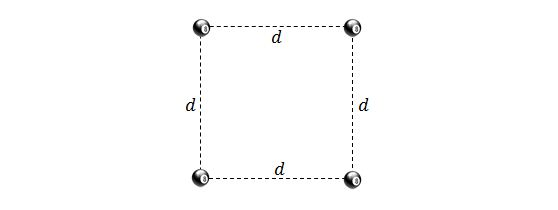Classical Mechanics

Gravitational Potential Energy

What is the approximate gravitational potential energy of the two-particle system, of masses $5.4\text{ kg}$ and $2.9 \text{ kg},$ separated by a distance of $15.0\text{ m}?$

Assumptions and details

• The universal gravitational constant is $G=6.67 \times 10^{-11} \text{ N}\cdot\text{m}^2\text{/kg}^2.$

Consider an $xy$-plane in deep space, where two particles A and B are located. Particle A is fixed at the origin and particle B is apart from particle A with a distance of $3.6\text{ m}.$ The masses of particles A and B are $24\text{ kg}$ and $12\text{ kg},$ respectively. If particle B is released from rest, what is the kinetic energy of B when it has moved $0.1\text{ m}$ toward A?

Assumptions and Details

• The universal gravitational constant is $G=6.67 \times 10^{-11} \text{ N}\cdot\text{m}^2\text{/kg}^2.$

The mean diameter of Mars is $6.7\times 10^3\text{ km}$ and its mass is $6.7\times 10^{23}\text{ kg}.$ What is the approximate escape speed on Mars?

Assumptions and Details

• The universal gravitational constant is $G=6.67 \times 10^{-11} \text{ N}\cdot\text{m}^2\text{/kg}^2.$Four identical particles are forming a square with side length $12.0\text{ m},$ as shown in the above figure. Then the side length of the square is reduced to $4.0\text{ m}.$ If the mass of each particles is $19.0\text{ kg},$ approximately how much gravitational potential energy of the four-particle system is reduced, assuming that the four particles are point particles?

Assumptions and Details

• The universal gravitational constant is $G=6.67 \times 10^{-11} \text{ N}\cdot\text{m}^2\text{/kg}^2 ?$

If a $6000 \text{ kg}$ space rocket is launched vertically from the surface of the Earth with initial energy $7.0 \times 10^{11} \text{ J},$ what will be the kinetic energy of the space rocket when it is $1.4 \times 10^7 \text{ m}$ from the center of the Earth, assuming that the mass and the radius of the Earth are $6.0 \times 10^{24}\text{ kg}$ and $6.4 \times 10^6 \text{ m},$ respectively?

Assumptions and Details

The universal gravitational constant is $G=6.67 \times 10^{-11} \text{ N}\cdot\text{m}^2\text{/kg}^2.$

×